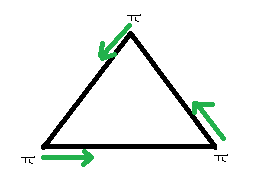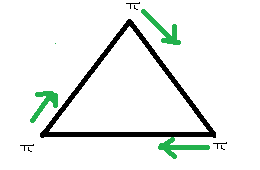# Puzzle 21 | (3 Ants and Triangle)

There are 3 ants sitting on three corners of a triangle. All ants randomly pick a direction and start moving along edge of the triangle. What is the probability that any two ants collide?

Hint: Every ant has two choices (pick either of two edges going through the corner on which ant is initially sitting).

Collision doesn’t happen only in following two cases
1) All ants move in counterclockwise direction.2) All ants move in clockwise direction.Since every ant has two choices (pick either of two edges going through the corner on which ant is initially sitting), there are total 23 possibilities.

Out of 23 possibilities, only 2 don’t cause collision. So, the probability of collision is 6/8 and the probability of non-collision is 2/8.